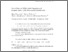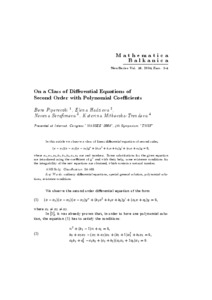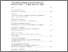# On a Class of Differential Equations of Second Order with Polynomial Coefficients

Piperevski, Boro and Hadzieva, Elena and Serafimova, Nevena and Mitkovska-Trendova, Katerina (2004) On a Class of Differential Equations of Second Order with Polynomial Coefficients. Mathematica Balkanica, New Series, 18 (3-4). pp. 411-418.Preview Text 3. MB-18-411-418.pdf Download (179kB) | PreviewPreview Text 3. mathbalkanica_info_toc_cont1834_pdf.pdf Download (40kB) | Preview

## Abstract

In this article we observe a class of linear differential equation of second order,
(x − x1)(x − x2)(x − x3)y” +(b2x2 + b1x + b0)y0 + (c1x + c0)y = 0,
where x1, x2, x3, b2, b1, b0, c1, c0 are real numbers. Some substitutions for the given equation are introduced using the coefficient of y00 and with their help, some existence conditions for the integrability of the new equations are obtained, which contain a natural number.

Item Type: Article Natural sciences > Matematics Faculty of Electrical Engineering Katerina Trendova 28 Jan 2015 08:41 28 Jan 2015 08:41 https://eprints.ugd.edu.mk/id/eprint/11929View Item